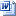## Display of grades and method of calculating the final grade for Test 1 – Evaluation of fundamental and specialized knowledge within the bachelor’s exam, September 2023 session

The results obtained in test 1 will be published on the website of the faculty at the address https://www.cs.ubbcluj.ro/anunturi/anunturi-licenta-si-disertatie/ and through the AcademicInfo platform, and the method of calculating the final grade in test 1 was the following:

Two members of the evaluation committee evaluated each subject. They gave a full grade each, so that there was no difference of more than one point between the two grades. The grade of the subject is the arithmetic mean of the marks awarded. The scoring was done as follows:

Mathematics field

1. Specialization Mathematics: the arithmetic mean of the grades obtained in the three Mathematics subjects (Algebra, Mathematical Analysis, and Geometry);
2. Specialization Mathematics and Computer Science: 2/3 grade in Mathematics + 1/3 grade in Computer Science. The grade from the Mathematics subject was calculated as the arithmetic mean of the grades obtained in the three Mathematics subjects (Algebra, Mathematical Analysis, and Geometry);

Computer science field

1. Specialization Computer Science: the arithmetic mean of the grades obtained in the three subjects of Computer Science (Algorithmic and programming, Databases, Operating systems).

SUBMISSION OF APPEALS

Appeals to test 1 can be submitted by graduates on September 6, 2023, between 9:00 a.m. and 11:00 a.m. at the address: https://forms.microsoft.com/e/RStQgU2chtFormular contestatie licenta 2023